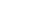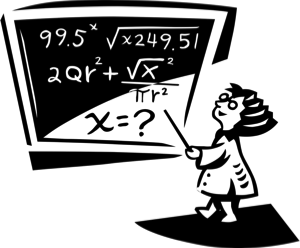• # Welcome!

Open or CloseThis section provides you with video self-tutorials in the areas of Algebra. I include what is known as Elementary Algebra, slowly move up to Intermediate Algebra and finally to what is considered College Algebra or even Pre-Calculus. It is equivalent to what is taught in high schools and colleges.

To get the most out of these Lessons, you are HIGHLY recommended to first review the
FREE Basic Math/Pre-Algebra lessons provided on this web site. Doing well in Algebra depends on knowing what I teach in those Lessons.

Both sample and a couple free complete video lessons are featured here so you can have a good idea of what is being done in each Lesson. Visit my
DVDs or Video-On-Demand pages to purchase complete (“premium”) lessons.

Premium Lessons may also be rented on YouTube. These rentals are for 72 hours. Links are provided with each lesson below.

*Special Note to Teachers, Parents and Tutors: If you plan to use my videos for teaching/tutoring purposes, you need to buy the DVDs (not just watch the free videos on this page with your students). The good news is no additional school licenses are needed!

Students: Although not required, it is appreciated if you could buy at least one DVD lesson from me. If you cannot do this, then perhaps you can make a small donation to my YouTube Channel.

Your purchases/tips allow me to dedicate more time to creating even MORE and BETTER videos for you! Thank you in advance!

NOTE: The videos have associated PDF Math Notes or Worksheets. Visit my Printshop for details.
• # Introduction to Algebra

Open or Close
Algebra: Introduction To Algebra

[NOTE: This video is only the first 11 minutes of the full lesson]

This lesson consists of providing you with a Self-Tutorial on what is algebra, what are variables, constants, coefficients, terms, and expressions. The tutor explains the use of proper notation, how to combine like terms, find the negation of an algebraic expression, how to evaluate an expression (by hand and by using your calculator), and finally, there is a VERY detailed section on how to translate English phrases into algebraic expressions.

PDF Math Notes: \$4.99
Video On Demand: \$9.99
DVD: \$19.99
• # Formulas From Geometry

Open or Close
Algebra: Formulas From Geometry

This FREE lesson consists of providing you with a basic review of the formulas from geometry you will most likely encounter in algebra (and other math classes like trigonometry and calculus). This is NOT a detailed, formal lesson.

Formulas and concepts covered include:

Angles (complementary & supplementary)
Triangles (perimeter and area)
Isosceles triangle
Equilateral triangle
Right triangle
The Pythagorean Theorem
Similar triangles
Perimeter and area formulas for Quadrilaterals (square, rectangle, parallelogram, trapezoid). Some diagonal formulas too, where applicable.
Cube (diagonal of face, diagonal of cube, surface area and volume)
Rectangular box [or Rectangular Parallelepiped] (diagonal of box, surface area and volume)
Prisms (Volume)
Pyramids (Volume)
Cone (slant height, lateral surface area, total surface area, volume)
Cylinder (lateral surface area, total surface area, volume)
Sphere (surface area and volume)
• # Greek Alphabet

Open or Close
Greek Alphabet

This
FREE video is to help students in math and science classes learn the Greek alphabet. The pronunciation is the English way of saying the letters in an American accent.

It is for use by math and science students and is NOT meant for those actually trying to learn Greek as a foreign language.

Get the extended Video Flash Cards for this lesson
HERE.

Get the Worksheet for this lesson
HERE.
• # Solving Linear Equations - Part 1: The Basics

Open or Close
Algebra: Solving Linear Equations - Part 1: The Basics

[NOTE: This video is only the first 18 minutes of the full lesson]

Lesson consists of providing you with a Self-Tutorial on how to solve linear equations. Tutor discusses what is an Equation, Solution, Solution Set, Equivalent Equations, Identity, Contradictions, Conditional Equations, Linear Equations, the Standard Form of a Linear Equation. I show you how to actually SOLVE linear equations going step-by-step. I don't skip steps. I review some of the properties of Equality here too. I remind you to check the work done and how to solve linear equations using a calculator. At the end, you will know how to easily solve equations that look like:

2[y - (4y - 1)] = 5 - 9y

No word problems are done here. This is shown in DETAIL in Part 2 of the Lesson.

PDF Math Notes: \$4.99
Video On Demand: \$9.99
DVD: \$19.99
• # Solving Linear Equations - Part 2: Applications [Sample 1]

Open or Close
Algebra: Solving Linear Equations - Part 2: Applications

[NOTE: This video is only the first 20 minutes of the full lesson]

This lesson consists of providing you with a Self-Tutorial on how to solve typical linear word problems (story problems or applied problems). The tutor shows you how to solve for a specific variable in formulas. He also discusses how to covert a repeating decimal into a fraction (which was skipped in Basic Math: Lesson 6 -"Fractions") and will teach you how to convert units of measurement.

Examples of word problems done include:

Finding a number based on certain criteria.
Word problems involving some geometry (triangle, rectangle, circle).
Age problems.
Mixture problems.
Money problems (story of my life!).
Rate-Time-Distance problems.
Percent Equations/problems.
Ratio and Proportion (concepts and solving problems, including similar triangles).
Problems dealing with Unit Price.

Video Rental (on YouTube): \$2.99 • 2nd Part of Rental HERE (\$1.99)
PDF Math Notes: \$4.99
Video On Demand: \$13.32 and \$6.67
DVD: \$29.99
• # Solving Linear Equations - Part 2: Applications [Sample 2]

Open or Close
Algebra: Solving Linear Equations - Part 2: Applications

[NOTE: This video is a 12 minute sample of the full lesson]

See link above for video details.
• # Solving Linear Inequalities [Sample 1]

Open or Close
Algebra: Solving Linear Inequalities

[NOTE: This video is only the first 23 minutes of the full lesson]

This lesson consists of providing you with a Self-Tutorial on how to solve typical linear inequalities of the form: ax + b > c (any of these symbols: <, >, ≤, ≥ can be used). The tutor goes over how to draw number line graphs. Also covered is the Double Inequality (a < x < b). Properties of Inequalities are reviewed. Answers are presented in Inequality Notation, Interval Notation, and Set Notation. Compound Linear Inequalities are solved and graphed. Finally, English Phrases are translated into inequalities and some Word Problems are solved.

Video Rental (on YouTube): \$4.99 • 2nd Part of Rental HERE (\$1.99)
PDF Math Notes: \$4.99
Video On Demand: \$13.32 and \$5.00
DVD: \$24.99
• # Solving Linear Inequalities [Sample 2]

Open or Close
Algebra: Solving Linear Inequalities

[
NOTE: This video is a 2 minute sample of the full lesson]

See link above for video details.
• # Graphing Linear and Double Inequalities

Open or Close
Graphing Linear and Double Inequalities

This is a
FREE 14 minute sample of my "Algebra: Solving Linear Inequalities" lesson. I go over how to draw number line graphs of inequalities. Also covered is the Double Inequality.
• # How to Solve One-Step Equations

Open or Close
How to Solve One-Step Equations

This is 2 of 4 videos I custom made for an educator in California for an experimental 1-week video homework program. I have only performed minor editing (so a few comments I make in videos *may* be out of context). Although not designed for the general public, I figured you may find them useful.

This video provides a quick lesson on how to solve one-step linear equations.
• # How to Solve Two-Step Equations

Open or Close
How to Solve Two-Step Equations

This is 3 of 4 videos I custom made for an educator in California for an experimental 1-week video homework program. I have only performed minor editing (so a few comments I make in videos *may* be out of context). Although not designed for the general public, I figured you may find them useful.

This video provides a quick lesson on how to solve two-step linear equations.
• # How to Solve Multi-Step Equations

Open or Close
How to Solve Multi-Step Equations

This is 4 of 4 videos I custom made for an educator in California for an experimental 1-week video homework program. I have only performed minor editing (so a few comments I make in videos *may* be out of context). Although not designed for the general public, I figured you may find them useful.

This video provides a quick lesson on how to solve multi-step linear equations.